Reach Us+44-7482-875032
Upper and Lower Weaky mX - andalpha;andpsi; - Continuous Multifunctions | OMICS International
Journal of Applied & Computational Mathematics
All submissions of the EM system will be redirected to Online Manuscript Submission System. Authors are requested to submit articles directly to Online Manuscript Submission System of respective journal.

# Upper and Lower Weaky mX - αψ - Continuous Multifunctions

M. Parimala*

Department of Mathematics, Bannari Amman Institute of Technology, Sathyamangalam-638401, Tamil Nadu, India

*Corresponding Author:
M. Parimala
Department of Mathematics, Bannari Amman Institute of Technology
Sathyamangalam-638401, Tamil Nadu, India
E-mail: [email protected]

Received Date: March 16, 2012; Accepted Date: May 14, 2012; Published Date: May 18, 2012

Citation: Parimala M (2012) Upper and Lower Weaky mX - αψ - Continuous Multifunctions. J Appl Computat Math 1:107. doi: 10.4172/2168-9679.1000107

Copyright: © 2012 Parimala M. This is an open-access article distributed under the terms of the Creative Commons Attribution License, which permits unrestricted use, distribution, and reproduction in any medium, provided the original author and source are credited.

Visit for more related articles at Journal of Applied & Computational Mathematics

#### Keywords

Minimal structure; mX -αψ -closed set; mX -αψ -continuous functions in minimal structure spaces

#### AMS (2000) Subject Classification

54A05, 54A20, 54C08, 54D10, 54C60.

#### Introduction

In 1961, Levine  introduced the notion of weakly continuous functions. Popa and Smithson [2,3] independently introduced the concept of weakly continuous multifunctions. Noiri  introduced the concept of minimal structure on a nonempty set. Also they introduced the notion of mX -open set and mX -closed set and characterize those sets using mX -cl and mX -int operators respectively. Further they introduced m-continuous functions  and studied some of its basic properties. Noiri and Popa  introduced and studied other forms of continuous multifunctions namely, slightly m-continuous multifunctions.

In this paper, we introduce mX -αψ -closed set and also we study some of the upper/lowermX -αψ -continuous multifunctions as the multifunctions are defined between a set satisfying certain minimal condition into a topological space. We obtain some characterizations and some properties of such multifunctions.

#### Preliminaries

In this section, we introduce the m-structure and define some important subsets associated to the m-structure and the relation between them.

Definition

Let X be a nonempty set and let mX ⊆ P(X) , where P(X) denote the power set of X . Where mX is an m-structure (or a minimal structure) on X , if φ and X belong to mX

The members of the minimal structure mX are called mX -open sets, and the pair (X,mX ) is called an m -space. The complement of mX -open set is said to be mX -closed.

Definition

 Let X be a nonempty set and mX an m -structure on X . For a subset A of X , mX -closure of A and mX -interior of A are defined as follows:

1. mX - Cl(A) =∩{F : A ⊆ F,X − F∈mX}

2. mX - Int(A) =∪{F :U ⊆ A,U ∈mX} .

Lemma

 Let X be a nonempty set and mX an m -structure on X . For subsets A and B of X , the following properties hold:

1. mX - Cl(X − A) = X −mX - Int(A) and mX - Int(X − A) = X −mX ) - Cl(A) .

2. If (X − A)∈mX , then mX - cl(A) = A and if A∈mX then mX - int(A) = A .

3. mX - Cl(φ ) =φ , mX - Cl(X) = X , mX - int(φ ) =φ and mX - int(X) = X .

4. If A ⊆ B then mX - Cl(A)⊆mX - Cl(B) and mX - int(A)⊆mX - int(B) .

5. A ⊆mX - Cl(A) and mX - Int(A)⊆ A .

6. mX - Cl(mX - Cl(A)) =mX - Cl(A) and mX - Int(mX - Int(A)) =mX - Int(A) .

Lemma

 Let (X,mX ) be an m -space and A a subset of X . Then x∈mX - cl(A) if and only if U ∩A ≠φ for every U ∈mX containing x .

Definition

 A minimal structure mX on a nonempty set X is said to have the property β if the union of any family of subsets belonging to mX belongs to mX .

Remark

 A minimal structure mX with the property β coincides with a generalized topology on the sense of Lugojan.

Lemma

 Let X be a nonempty set and mX an m -structure on X satisfying the property β . For a subset A of X , the following property hold:

1. A∈mX iff mX - int(A) = A

2. A∈mX iff mX - cl(A) = A

3. mX - int(A)∈mX and mX - cl(A)∈mX

Definition

A subset A of an m -space (X,mX ) is called

1. an mX -preopen set  if A ⊆U - int(mX - cl(A)) and a mX -preclosed set if mX - cl(mX - int(A))⊆ A ,

2. an mX -semiopen set  if A ⊆mX - cl(mX - int(A)) and a mX -semiclosed set if mX - int(mX - cl(A))⊆ A ,

3. an mX -semi generalized-closed  (briefly mX - sg -closed) set if mX - scl(A)⊆U whenever A ⊆U and U is mX -semi-open in (X,mX ) . The complement of an mX - sg -closed set is called an mX - sg -open set.

The mX -pre closure (resp. mX -semi closure, mX -α -closure) of a subset A of an m-space (X,mX ) is the intersection of all mX -pre closed (resp. mX -semi closed, mX -α -closed) sets that contain A and is denoted by mX - pcl(A) (resp. mX - scl(A) , mX -mX ).

#### mX -αψ -closed and mX -αψ -open sets

Definition

A subset A of an m -space (X,mX ) is called an

1. mX -α -open set if A ⊆mX - int(mX - cl(mX - int(A))) and an mX -α -closed set if mX - cl(mX - int(mX - cl(A)))⊆ A ,

2. mX -ψ -closed set if mX - scl(A)⊆U whenever A ⊆U and U is mX - sg -open in (X,mX ) .The complement of an mX -ψ -closed set is called an mX -ψ -open set.

3. mX -αψ -closed set if mX -ψ cl(A)⊆U whenever A ⊆U and U is mX -α -open in (X,mX ) .The complement of an mX -αψ -closed set is called an mX -αψ -open set.

Notation

For an m -space (X,mX ) , O(X,mX ) (resp. SO(X,mX ) , PO(X,mX ) , αO(X,mX ) , SGO(X,mX ) , ψ O(X,mX ) , αψ - O(X,mX ) ) denotes the class of all open (resp. mX -semiopen, mX -preopen, mX -α -open, mX -sg-open, mX -ψ -open, mX -αψ -open) subsets of (X,mX ) .

Definition

Let (X,mX ) be an m -space and let A be a subset of X . Then

1. the intersection of all mX -mX -closed sets containing A is called the mX -αψ -closure of A and is denoted by mX -αψ - cl(A) .

2. the union of all mX -αψ -open sets that are contained in A is called the mX -αψ -interior of A and is denoted by mX -αψ - int(A)

Example (1)

Let X = {a,b,c,d} . Define the m -structure on X as follows: mX = {φ ,X,{a},{b},{a,b} .

Then SO(X,mX ) = {φ ,X,{a},{b},{a,c}, {a,d},{b,d},{a,c,d}} ,

αO(X,mX ) = {φ ,X,{a},{b},{a,b},{a,c},{a,b,c}} and

αψ -O(X,mX ) = {φ ,X,{a},{b},{c},{a,b},{a,c},{a,d},{b,c},{b,d},{a,b,c},{a,c,d}} .

Example (2)

Let X = {a,b,c} . Define the m -structure on X as follows: mX = {φ ,X,{a},{b}} .

Then SO(X,mX ) = {φ ,X,{a},{b},{a,c},{b,c}} ,

αO(X,mX ) = {φ ,X,{a},{b}} and αψ -O(X,mX ) = P(X) .

Example (3)

Let X = {a,b,c,d} . Define the m -structure on X as follows: mX = {φ ,X,{a},{b},{a,b,c},{a,b,d}} .

Then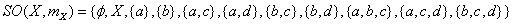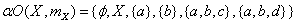and αψDefinition

The intersection of all mX -α -open subsets of (X,mX ) containing A is called the mX -α -kernel of A (briefly, mX -αker(A) ) i.e. mX -α ker(A) =∩{G∈mX -αO(X) : A ⊆G} . And mX - s ker(A) , mX - sgker(A) , an mX -ψ ker(A) are defined similarly.

Theorem (1)

Let A be a subset of (X,mX ) , then A is mX - (α ,ψ ) -closed if and only if mX -ψ cl(A)⊆mX -αker(A) .

Proof

Suppose that A is mX -αψ -closed and let D = {S : S ⊆ X,A⊆ S : Sis anmX -α -open}. Then mX -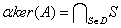Observe that S∈D implies that A ⊆ S follows mX -ψ cl(A)⊆ S for all S∈D .

Conversely, if mX -ψ cl(A)⊆mX - aker(A) , take S∈αO(X,mX ) such that A ⊆ S then by hypothesis,

mX -ψ cl(A)⊆mX -αker(A)⊆ S .

This shows that A is mX -αψ -closed.

Theorem (2)

For subsets A and B of (X,mX ) , the following properties hold:

1. If A is mX -ψ -closed, then A is mX -αψ -closed.

2. If mX has the property β and A is mX -αψ -closed and mX - α -open then A is mX -ψ -closed.

3. If A is mX -αψ -closed and A ⊆ B ⊆ψ cl(A) then B is mX - αψ -closed.

Proof

1. Let A be an mX -ψ -closed set in (X,mX ) . Let A ⊆U , where U is mX -α -open in (X,mX ) . Since A is mX -ψ -closed, mX - ψ cl(A) = A , mX -ψ cl(A)⊆U . Therefore, A is mX -αψ -closed.

2. Since A is mX -α -open and mX -αψ -closed, we have mX - ψ cl(A)⊆ A . Therefore, A is mX -ψ -closed

3. Let U be an mX -α -open set of (X,mX ) such that B ⊆U , then A ⊆U . Since A is mX -αψ -closed, mX -ψ cl(A)⊆U . Now mX -ψ cl(B)⊆mX -ψ cl(mX -ψ cl(A))⊆U . Therefore, B is also an mX -αψ -closed set of (X,mX ) .

Theorem (3)

Union of two mX -αψ -closed sets is mX -αψ -closed.

Proof

Let A and B be two mX -αψ -closed sets in (X,mX ) . Let A∪B ⊆U , U is mX -α -open. Since A and B are mX -αψ -closed sets, mX -ψ cl(A)⊆U and mX -ψ cl(B)⊆U . This implies that mX - ψ cl(A∪B)⊆mX -ψ cl(A) -ψ cl(B)⊆U and so mX -ψ cl(A∪B)⊆U . Therefore A∪B is mX -αψ -closed.

Theorem (4)

Let mX be an m -structure on X satisfying the property β and A ⊆ X . Then A is an mX -αψ -closed set if and only if there does not exist a nonempty mX -α -closed set F such that F ≠φ and F ⊆mX - ψ cl(A)− A .

Proof

Suppose that A is an mX -αψ -closed set and let F ⊆ X be an mX -α -closed set such that F ⊆mX -ψ cl(A)− A . It follows that, A ⊆ X − F and X − F is an mX -α -open set. Since A is an mX - αψ -closed set, we have that mX -ψ cl(A)⊆ X − F and F ⊆ X −mX -ψ cl(A) . Follows that, F+(V)⊂mX -ψ cl(A)∩(X −mX -ψ cl(A)) =φ , implying that F =φ .

Conversely, if A ⊆U and U is an mX -α -open set, then mX - ψ cl(A)∩(X −U)⊆mX -ψ cl(A)∩(X − A) =mX -ψ cl(A)− A . Since mX -ψ cl(A)− A does not contain subsets mX -α -closed sets different from the empty set, we obtain that mX -ψ cl(A)∩(X −U) =φ and this implies that mX -ψ cl(A)⊆U in consequence A is mX -αψ -closed.

We can observe that if in Theorem 3.11., the property β is omitted then the result can be false as we can see in the following example.

Example

Let X = {a,b,c,d} . The m -structure on X is defined as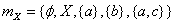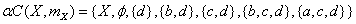and αψ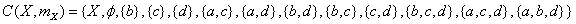The set {a} is not an mX -αψ -closed set and there does not exist mX -α -closed set F such that F ≠φ and F ⊆mX -ψ cl(A)− A .

Theorem (5)

Let (X,mX ) be an m -space and A ⊆ X , then A is mX -αψ -open if and only if F ⊂mX -ψ int(A) where F is mX -α -closed and F ⊂ A .

Proof

Let A be an mX -αψ -open, F be mX -α -closed set such that F ⊂ A . Then X − A ⊂ X − F , but X − F is mX -α -closed and X − A is mX -αψ -closed implies that mX -ψ cl(X − A)⊂ X − F . Follows that X −mX -ψ int(A)⊂ X − F . In consequence F ⊂mX -ψ int(A) .

Conversely, if F is mX -α -closed, F ⊂ A and F ⊂mX -ψ int(A) . Let X − A ⊂U where U is mX -α -open, then X −U ⊂ A and X −U is mX -α -closed. By hypothesis, X −U ⊂mX -ψ int(A) . Follows X −mX -ψ int(A)⊂U but it is equivalent to mX -ψ cl(X − A)⊂U . Therefore, X − A is mX -αψ -closed and hence A is mX -αψ -open.

#### Weak mX -αψ -continuous and almost mX -αψ-continuous multifunctions

Definition (1)

Let (X,mX ) be an m -space and (Y,σ ) a topological space. A multifunction F : (X,mX )→(Y,σ ) is said to be

1. upper mX -αψ -continuous (resp. upper almost mX -αψ -continuous, upper weakly mX -αψ -continuous) at a point x if for each open set V of Y containing F(x) , there exists an mX - αψ -open set U of mX containing x such that F(U)⊂V (resp. F(U)⊂int(cl(V)),F(U)⊂ cl(V)) ,

2. lower mX -αψ -continuous (resp. lower almost mX -αψ -continuous, lower weakly mX -αψ -continuous) at a point x∈X if for each open set V of Y such that F(x)∩V ≠φ , there exists an mX -αψ -open set U of mX containing x such that F(u)∩V ≠φ (resp. F(u)∩int(cl(V)) ≠φ ,F(u)∩cl(V) ≠φ ) for each u∈U ,

3. upper/lower mX -αψ -continuous (resp. almost mX -αψ -continuous, weakly mX -αψ -continuous) if it has this property at each point x∈X .

Definition (2)

multifunction F : (X,mX )→(Y,σ ) is said to be almost mX -αψ -open if F(U)⊂int(cl(F(U))) for every mX -αψ -open set U of mX .

Theorem (1)

If a multifunction F : (X,mX )→(Y,σ ) is upper weakly mX -αψ -continuous and almost mX -αψ -open, then F is upper almost mX -αψ -continuous.

Proof

Let V be any open set in Y containing F(x) . Then there exists an mX -αψ -open set U of mX containing x such that F(U)⊂ cl(V) . Since F(x) is almost mX -αψ -open, F(U)⊂int(cl(F(U)))⊂int(cl(V)) . Therefore, F is upper almost mX -αψ -continuous.

Theorem (2)

Let F : (X,mX )→(Y,σ ) be a multifunction such that F(x) is open in Y for each x∈X . Then, the following properties are equivalent:

1. F is lower mX -αψ -continuous;

2. F is lower almost mX -αψ -continuous;

3. F is lower weakly mX -αψ -continuous.

Proof

(i) ⇒ (ii) and (ii) ⇒ (iii): The proofs of these implications are obvious.

iii) ⇒ (i): Let x∈X and V be any open set such that F(x)∩V ≠φ . There exists an mX -αψ -open set U of mX such that F(u)∩cl(V) ≠φ for each u∈U . Since F(u) is open, F(u)∩V ≠φ for each u∈U and hence F is lower mX -αψ -continuous.

#### Slightly mX -αψ -continuous Multifunctions

Definition

Let (X,mX ) be an m -space and (Y,σ ) a topological space. A multifunction F : (X,mX )→(Y,σ ) is said to be

1. upper slightly mX -αψ -continuous if for each A∈mX and each clopen set V of Y containing F(x) , there exists an mX -αψ -open set U of mX containing x such that F(U)⊂V ,

2. lower slightly mX -αψ -continuous if for each x∈X and each clopen set V of Y such that F(x)∩V ≠φ , there exists an mX -αψ -open set U of mX containing x such that F(u)∩V ≠φ for each u∈U .

Theorem (1)

For a multifunction F : (X,mX )→(Y,σ ) , the following are equivalent:

1. F is upper slightly mX -αψ -continuous;

2. F+(V) =mX -αψ - int(F(V)) for each V ∈CO(Y) ;

3. F(V)) -αψ - cl(F−(V)) for each V ∈CO(Y) .

Proof

(i) ⇒ (ii): Let V be any clopen set of Y and x∈F+(V) . Then F(x)∈V . There exists an mX -αψ -open set U of mX containing x such that F(U)⊂V . Thus x∈U ⊂ F+(V) and hence x∈mX - αψ - int(F+(V)) . Therefore, we have F+(V)⊂mX -αψ - int(F+(V)) . But , mX -αψ - int(F+(V))⊂ Fv(V) , we obtain F+(V) =mX -αψ - int(F+(V)) .

(ii) ⇒ (iii): Let K be any clopen set of Y . Then Y −K is clopen in Y . By (ii) and Lemma 2.3., we have X − F+(K) = (Y −K) =mX - αψ - int(F+(Y −K)) = X −[mX -αψ - cl(F−(K))] . Therefore, we obtain F-(K) =mX -αψ - cl(F-(K)) .

(iii) ⇒ (ii): This follows from the fact that F-(Y − B) = F+(B) for every subset B of Y .

(ii) ⇒ (i): Let x∈X and V be any clopen set of Y containing F(x) . Then x∈F+(V) =mX -αψ - int(F+(V)) . There exists an mX - αψ -open set U of mX containing x such that x∈U ⊂ F+(V) . Therefore, we have x∈U , U is an mX -αψ -open set of mX and f (U)⊂V. Hence F is upper slightly mX -αψ -continuous.

Theorem (2)

For a multifunction F : (X,mX )→(Y,σ ) , the following are equivalent:

1. F is lower slightly mX -αψ -continuous;

2. F-(V) =mX -αψ - int(F-(V)) for each V ∈CO(Y) ;

3. F+(V) =mX -αψ - cl(F+(V)) for each V ∈CO(Y) .

Proof

(i) ⇒ (ii): Let V ∈CO(Y) and x∈F-(V) . Then F(x)∩V ≠φ and by (i) there exists an mX -αψ -open set U of mX containing x such that F(u)∩V ≠φ for each u∈U . Therefore, we have U ⊂ F-(V) and hence x∈U ⊂mX -αψ - int(F-(V)) . Thus, we obtain F-(V)⊂mX - αψ - int(F-(V)) and by Lemma 2.3., F-(V) =mX -αψ - int(F-(V)) .

(ii) ⇒ (iii): Let V ∈CO(Y) . Then Y −V ∈CO(Y) and by (ii) we have X − F+(V) = F-(Y −V) =mX -αψ - int(F-(Y −V)) = X −mX - αψ - cl(F+(V)) . Hence we obtain F+(V) =mX -αψ - cl(F+(V)) .

(iii) ⇒ (i): Let x be any point of X and V any clopen set of Y such that F(x)∩V ≠φ . Then x∈F−(V) and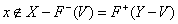. By (iii), we have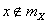-αψ - cl(F+(Y −V)) . By Lemma 2.4., there exists an mX -αψ -open set of mX containing x such that U ∩F+(Y −V) =φ , hence U ⊂ F-(V) . Therefore, F(u)∩V ≠φ for each u∈U and F is lower slightly mX -αψ -continuous.

Corollary (1)

For a multifunction F : (X,mX )→(Y,σ ) , where mX has the property β , the following are equivalent:

1. F is upper slightly mX -αψ -continuous;

2. F+(V) is mX -αψ -open in (X,mX ) for each V ∈CO(Y) ;

3. F-(V) is mX -αψ -closed in (X,mX ) for each V ∈CO(Y) .

Corollary (2)

For a multifunction F : (X,mX )→(Y,σ ) , where mX has the property β , the following are equivalent:

1. F is lower slightly mX -αψ -continuous;

2. F-(V) is mX -αψ -open in (X,mX ) for each V ∈CO(Y) ;

3. F+(V) is mX -αψ -closed in (X,mX ) for each V ∈CO(Y) .

#### References

Select your language of interest to view the total content in your interested language

### Article Usage

• Total views: 11909
• [From(publication date):
May-2012 - Aug 22, 2019]
• Breakdown by view type
• HTML page views : 8116
• PDF downloads : 3793

## Post your commentCan't read the image? click here to refresh
###### Peer Reviewed Journals

Make the best use of Scientific Research and information from our 700 + peer reviewed, Open Access Journals

International Conferences 2019-20

Meet Inspiring Speakers and Experts at our 3000+ Global Annual Meetings

Top